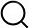# Also in the Article

Procedure

Descriptive statistics were used to describe the patient's baseline characteristics. The continuous data were presented as mean ± standard deviation (SD) or median and percentiles (interquartile range [IQR]), while categorical data were expressed as numbers of subjects and percentages. To analyze the difference between two groups of patients, independent t-tests and Mann-Whitney U test were used to compare the continuous data. The chi-square test or Fisher's exact test was used for categorical data. The inhospital mortality rates were compared using Kaplan–Meier method with the log-rank test or Peto-Peto test. Using the Cox proportional hazard model, the predictors for inhospital mortality and survival were analyzed by univariate analysis, and the multivariate analysis included only the variables with a p value of less than 0.2 from the univariate analysis. A p value of less than 0.05 was considered to be statistically significant. All statistical analyses were performed using the R program version 3.6.3.

Note: The content above has been extracted from a research article, so it may not display correctly.

Q&A